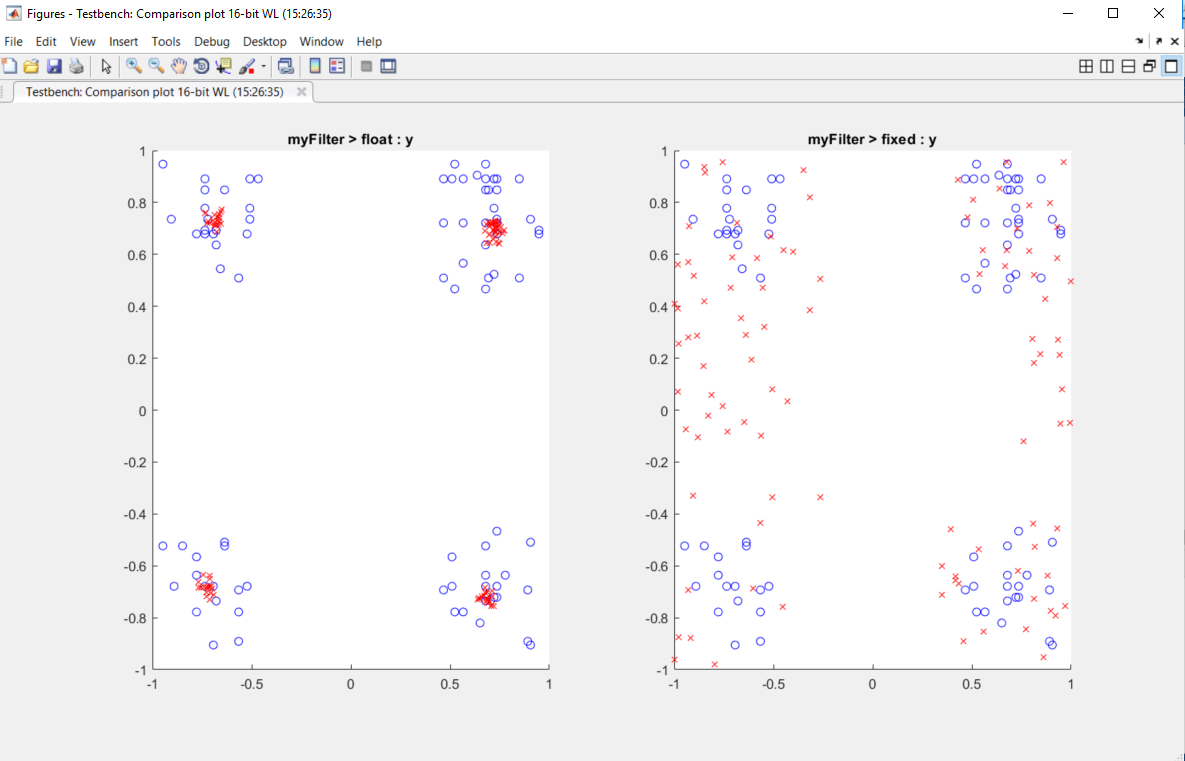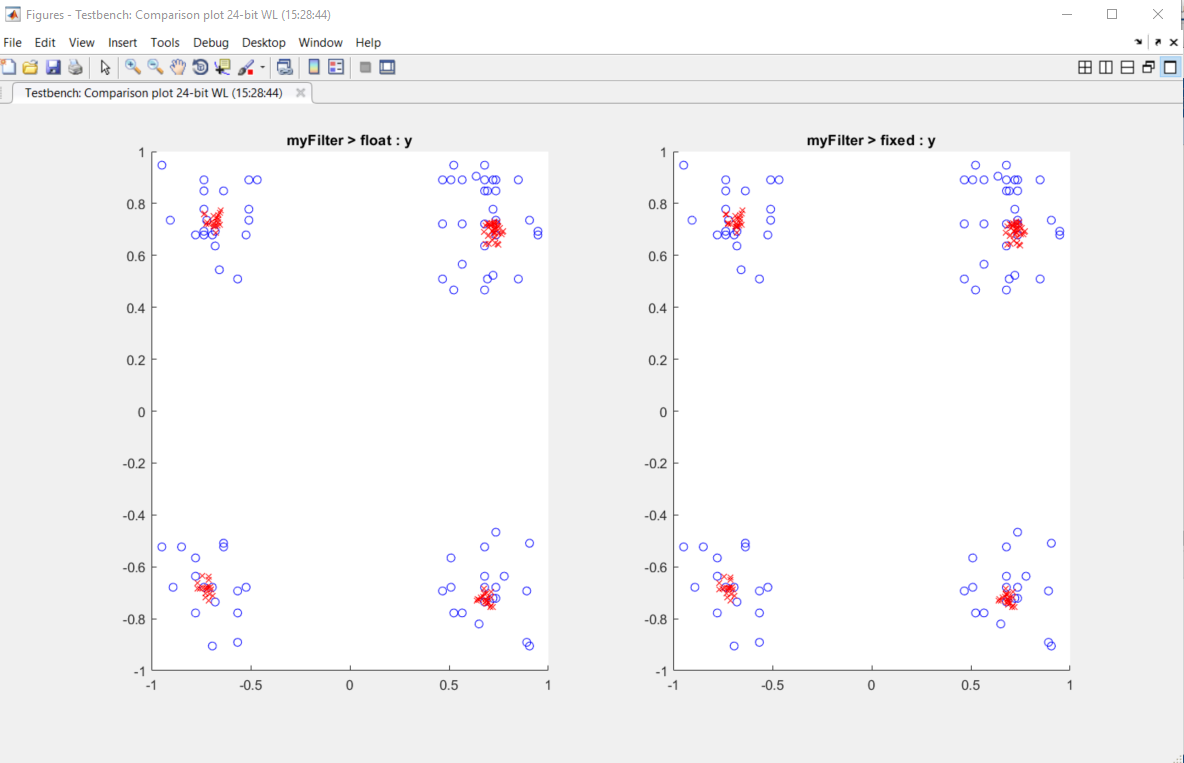## Visualize Differences Between Floating-Point and Fixed-Point Results

To complete this example, you must install the following products:

• MATLAB®

• Fixed-Point Designer™

• C compiler

You can use `mex -setup` to change the default compiler. See Change Default Compiler.

### Inspect Example Files

TypeNameDescription
Function code`myFilter.m`Entry-point MATLAB function
Test file`myFilterTest.m`MATLAB script that tests `myFilter.m`
Plotting function`plotDiff.m`Custom plot function
MAT-file`filterData.mat`Data to filter.

### Set Up Configuration Object

1. Create a `coder.FixptConfig` object.

`fxptcfg = coder.config('fixpt');`
2. Specify the test file name and custom plot function name. Enable logging and numerics testing.

```fxptcfg.TestBenchName = 'myFilterTest'; fxptcfg.PlotFunction = 'plotDiff'; fxptcfg.TestNumerics = true; fxptcfg.LogIOForComparisonPlotting = true; fxptcfg.DefaultWordLength = 16; ```

### Convert to Fixed Point

Convert the floating-point MATLAB function, `myFilter`, to fixed-point MATLAB code. You do not need to specify input types for the `codegen` command because it infers the types from the test file.

`codegen -args {complex(0, 0)} -float2fixed fxptcfg myFilter`

The conversion process generates fixed-point code using a default word length of `16` and then runs a fixed-point simulation by running the `myFilterTest.m` function and calling the fixed-point version of `myFilter.m`.

Because you selected to log inputs and outputs for comparison plots and to use the custom plotting function, `plotDiff.m`, for these plots, the conversion process uses this function to generate the comparison plot.The plot shows that the fixed-point results do not closely match the floating-point results.

Increase the word length to `24` and then convert to fixed point again.

```fxptcfg.DefaultWordLength = 24; codegen -args {complex(0, 0)} -float2fixed fxptcfg myFilter```

The increased word length improved the results. This time, the plot shows that the fixed-point results match the floating-point results.